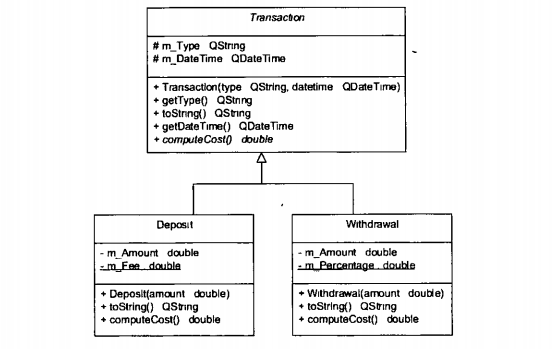# Cloud Stack Ninja

Consider the following UML class diagram:A user can make one of two transactions. A deposit or a withdrawal, then the transaction is added to a list of type QMap<...>. I made a class that manages the transactions like so:

``````#ifndef TRANSACTIONLIST_H
#define TRANSACTIONLIST_H
#include <QMap>
#include <QDateTime>
#include <QString>
#include <transaction.h> //should this be withdrawal.h and deposit.h?

class TransactionList
{
public:
~TransactionList();
QString toString() const;
private:
QMap<QDateTime, Transaction*> m_TransactionList;
};

#endif // TRANSACTIONLIST_H
``````

Assume I added the transactions correctly to the QMap. I want to implement the toString() method, in TransactionList, the returns the details of all transactions in the list. In the toString() method I want to call toString() of the correct derived class. For example, if a transaction is of type Deposit I want to call the toString() of Deposit. I know I can achieve this by casting the transaction type to (for example) Deposit but I'm sure there's a simpler way. Below is my toString() implementation but I got stuck when I thought about what I'm asking you here:

``````QString TransactionList::toString() const
{
QString str;
str.append("Transactions:\n");

QMapIterator<QDateTime, Transaction*> i(m_TransactionList);
while (i.hasNext()) {
i.next();
str.append(i.value()->toString());
}
}
``````

Thanks.

UPDATE:
Below are the implementations of the toString function in the superclass and bass classes:

``````QString Transaction::toString()
{
QString str;
str.append("Type: ");
str.append(getType());
str.append("\n");
str.append("Date and Time: ");
str.append(getDateTime().toString());

return str;
}
``````
``````QString Deposit::toString()
{
QString str;
str.append(Transaction::toString());
str.append("\nAmount: R");
str.append(QString::number(m_Amount));
str.append("\n");

return str;
}
``````
``````QString Withdrawal::toString()
{
return Transaction::toString() + "\nAmount: R" + QString::number(m_Amount) + "\n";
}
``````

I thought this might be relevant.